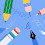-->

# Introduction of MATLAB Software for data visualization analysis

MATLAB (Matrix Laboratory) is a Engineering computing environment developed by MathWorks, Inc. for computing and visualizing data. MATLAB is an interactive system and as a programming language, the basic element of which is a data array (scalar, vector, matrix and double matrix). Besides matrix operations, MATLAB also offers the same programming features as other computing languages.

MATLAB can be executed on Windows and LINUX systems. The MATLAB software package contains many functions for data analysis. There are also several Toolboxes that can be used to expand the capabilities of MATLAB.

In this computational statistics article, we will discuss MATLAB version 9.4 2018. When starting MATLAB, a Command Window window will appear or it can be called the Command Window with a prompt that will be used to write command code. Normally, in MATLAB there are other windows besides the Command Window namely Workspace, Details folder and others.

WINDOW MATLAB

There are several windows (Window) in MATLAB. These windows include,

COMMAND WINDOW. The Command Window that I mentioned earlier that the Command Window is used to write command code that will produce numeric output or the results of logical analysis (IF Then), errors in typing in the Command Window and others. Where the Windows Command display is shown in the image below,

WORKSPACE MATLAB will always remind ENTER commands and all variable values ​​made. These variables are stored in the Workspace window. The variable can be called at any time by typing the name of the variable in the Command Window then ENTER. In MATLAB Temp, temp and TEMP represent different variables. Variables can be viewed and edited in a window like a spreadsheet by double-clicking on the variable name. Below is an example of a Workspace display.

COMMAND HISTORY. This window displays statements that are executed in the command window window. Command History can be used to view, copy and execute statements.

HELP. This window contains information about procedures for using the MATLAB command code. Code Description Commands that are on the HELP are standard MATLAB commands or toolboxes that have been installed.

FIGURE / GRAPHIC. This window will automatically appear to display images or graphics made by MATLAB. Where only one window figure can be opened at a time.

EDITOR / DEBUGGER. This window is used for making m-files. M-file is a collection of MATLAB code commands for a specific purpose. This window can be accessed using the pull-down menu, by selecting File ==> New ==> M-File.

As for how to get MATLAB r 2018 v9.4 software you can visit and download it at Agung Panduan

### 8 Responses to " Introduction of MATLAB Software for data visualization analysis"

1.2.I would state, you do the genuinely amazing.This substance is made to a wonderful degree well. weebly v wix

3.At that point you get the chance to stress over interfaces between the instruments, reports, security (both interior and outer), and the magnificent, unstoppable, unusual, and customarily annihilation propensities for the end clients even after you've given total start to finish preparing on the new item. ExcelR Data Science Courses

4.data science course

5.Data Science Course

6.Nice post thanks for sharing your information
Data Science Certification in Bangalore

7.Correlation vs Covariance
Simple linear regression

8.A debt of gratitude is in order for sharing the information, keep doing awesome... I truly delighted in investigating your site. great asset...
Data Science Course in Bangalore